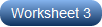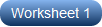1. Worksheets>
2. Math>
3. Pre-Algebra>
5. Add, Subtract, Multiply, and Divide

Set the ball rolling and practice this batch of printable radical operations worksheets to enrich your skills of performing arithmetic operations with radicals. Familiarize yourself with the various rules or laws that are applicable to adding, subtracting, multiplying, or dividing radicals while solving the problems in these pdfs. Comprising two levels of practice, this page presents radical expressions with two and three terms and involving like and unlike radicands. Click on the free worksheet and set start practice!

Simplifying Radical Expressions - Level 1

Get hold of these printable worksheets to hone your skills in simplifying radical expressions. The problems in these worksheets have radicals involving arithmetic operations.Simplifying Radical Expressions - Level 2

Crack the questions in this set of pdf worksheets that stand out with a higher level of difficulty. Factorize the radicands and write the radicals in the simplest form. Having obtained an expression with like radicands, proceed with the operations.Related Worksheets